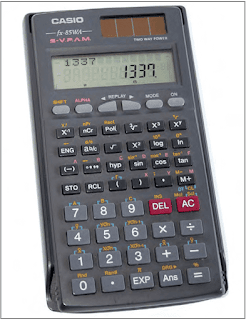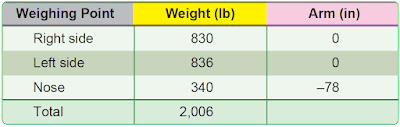# Electronic Calculator

Determining the CG of an airplane in inches for the main-wheel weighing points can be done with any simple electronic calculator that has addition (+), subtraction (–), multiplication (×), and division (÷) functions. [Figure 1] Scientific calculators with such additional functions as memory (M), parentheses (( )), plus or minus (+/–), exponential (yx), reciprocal (1/x), and percentage (%) functions allow more complex problems to be solved or simple problems to be solved using fewer steps.Figure 1. A typical electronic calculator is useful for solving most types of weight and balance problems

According to Figure 2, the weight of the nosewheel (F) is 340 pounds, the distance between main wheels and nosewheel (L) is –78 inches, and the total weight (W) of the airplane is 2,006 pounds. L is negative because the nosewheel is ahead of the main wheels.Figure 2. Weight and balance data of a typical nosewheel airplane

To determine the CG, use this formula:

Key the data into the calculator and press the equal (=) key.The answer should read as follows:

(340)(×)(78)(+/–)(÷)(2006)(=) –13.2

The arm of the nosewheel is negative, so the CG is –13.2 or 13.2 inches ahead of the main wheel weighing points.

RELATED POSTS